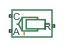# Translational Hydro-Mechanical Converter

(To be removed) Interface between hydraulic and mechanical translational domains

The Hydraulic library will be removed in a future release. Use the Isothermal Liquid library instead. (since R2020a)

## Library

Hydraulic Elements

•## Description

The Translational Hydro-Mechanical Converter block models an ideal transducer that converts hydraulic energy into mechanical energy, in the form of translational motion of the converter output member, and vice versa. The compressibility option makes the converter account for dynamic variations of the fluid density.

Using this block as a basic element, you can build a large variety of hydraulic cylinder models by adding application-specific effects, such as leakage, friction, hard stops, and so on.

The converter is simulated according to the following equations:

`$q=\frac{d\left(\frac{\rho }{{\rho }_{l}^{0}}V\right)}{dt}=\frac{d\left(\frac{\rho }{{\rho }_{l}^{0}}\right)}{dt}V+\frac{\rho }{{\rho }_{l}^{0}}\cdot \epsilon \cdot \left({v}_{R}-{v}_{C}\right)\cdot A$`
`$F=\epsilon \cdot p\cdot A$`

where

 q Flow rate to the converter chamber A Effective piston area vR Converter rod velocity vC Converter case velocity F Force developed by the converter p Gauge pressure of fluid in the converter chamber V Piston volume α Relative amount of trapped air ρl0 Fluid density at atmospheric conditions ρg0 Gas density at atmospheric conditions p0 Atmospheric pressure γ Specific heat ratio βl Bulk modulus at atmospheric conditions and no gas ε Mechanical orientation of the converter (`1` if increase in fluid pressure causes positive displacement of R relative to C, `-1` if increase in fluid pressure causes negative displacement of R relative to C)

The piston volume is computed according to

`$\begin{array}{l}V={V}_{dead}+A\cdot \left({x}_{0}+x\right)\\ \frac{dx}{dt}=\epsilon \cdot \left({v}_{R}-{v}_{C}\right)\end{array}$`

where

 Vdead Chamber dead volume x0 Piston initial position x Piston displacement from initial position

Port A is a hydraulic conserving port associated with the converter inlet. Ports R and C are translational mechanical conserving ports associated with the rod and the case of the converter, respectively.

The block dialog box does not have a Source code link. To view the underlying component source, open the following files in the MATLAB® editor:

• For incompressible converter implementation — `translational_converter_incompressible.ssc`

• For compressible converter implementation — `translational_converter_compressible.ssc`

## Basic Assumptions and Limitations

The block simulates an ideal converter, with an option to account for fluid compressibility. Other effects, such as hard stops, inertia, or leakage, are modeled outside of the converter.

## Parameters

Piston area

Effective piston area. The default value is `5e-4` m^2.

Converter orientation

Specifies converter orientation with respect to the globally assigned positive direction. The converter can be installed in two different ways, depending upon whether it exerts force in the positive or in the negative direction when pressure is applied at its inlet:

• ```Pressure at A causes positive displacement of R relative to C``` — Increase in the fluid pressure results in a positive displacement of port R relative to port C. This is the default.

• ```Pressure at A causes negative displacement of R relative to C``` — Increase in the fluid pressure results in a negative displacement of port R relative to port C.

Compressibility

Specifies whether fluid density is taken as constant or varying with pressure. The default value is `Off`, in which case the block models an ideal transducer. If you select `On`, the block dialog box displays additional parameters that let you model dynamic variations of the fluid density without adding any extra blocks.

Piston displacement

Select method to determine displacement of port R relative to port C:

• `Calculate from velocity of port R relative to port C` — Calculate displacement from relative port velocities, based on the block equations. This is the default method.

• `Provide input signal from Multibody joint` — Enable the input physical signal port p to pass the displacement information from a Multibody joint. Use this method only when you connect the converter to a Multibody joint by using a Translational Multibody Interface block. For more information, see How to Pass Position Information.

This parameter is enabled when Compressibility is `On`.

Initial piston position

Initial offset of the piston from the cylinder cap. This parameter is enabled when Compressibility is `On` and Piston displacement is ```Calculate from velocity of port R relative to port C```. The default value is `0` m.

Volume of fluid in the chamber at zero piston position. This parameter is enabled when Compressibility is `On`. The default value is `1e-4` m^3.

Specific heat ratio

Gas-specific heat ratio. This parameter is enabled when Compressibility is `On`. The default value is `1.4`.

Initial pressure

Initial pressure in the chamber. This parameter specifies the initial condition for use in computing the block's initial state at the beginning of a simulation run. It is enabled when Compressibility is `On`. The default value is `0`.

## Ports

The block has the following ports:

`A`

Hydraulic conserving port associated with the converter inlet.

`R`

Mechanical translational conserving port associated with the rod of the converter.

`C`

Mechanical translational conserving port associated with the case of the converter.

`P`

Physical signal input port that passes the position information from a Simscape™ Multibody™ joint. Connect this port to the position sensing port p of the joint. For more information, see Connecting Simscape Networks to Simscape Multibody Joints. To enable this port, set the Interface displacement parameter to `Provide input signal from Multibody joint`.

## References

 Manring, N.D., Hydraulic Control Systems, John Wiley & Sons, New York, 2005

 Meritt, H.E., Hydraulic Control Systems, John Wiley & Sons, New York, 1967

## Version History

Introduced in R2007a

expand all